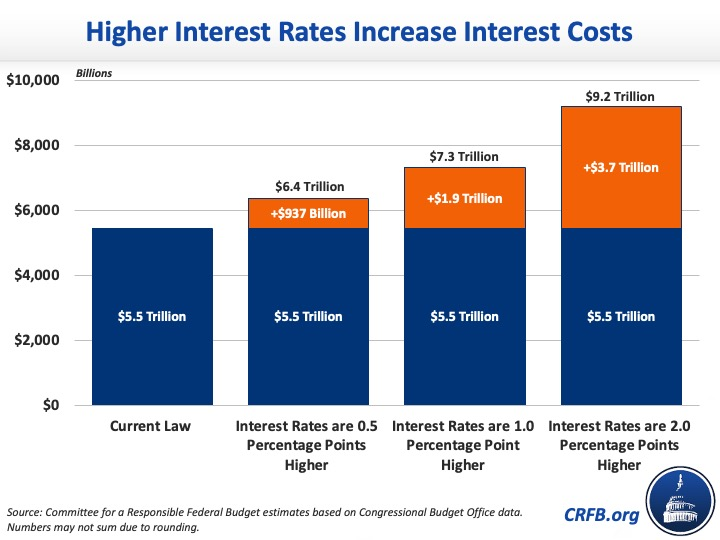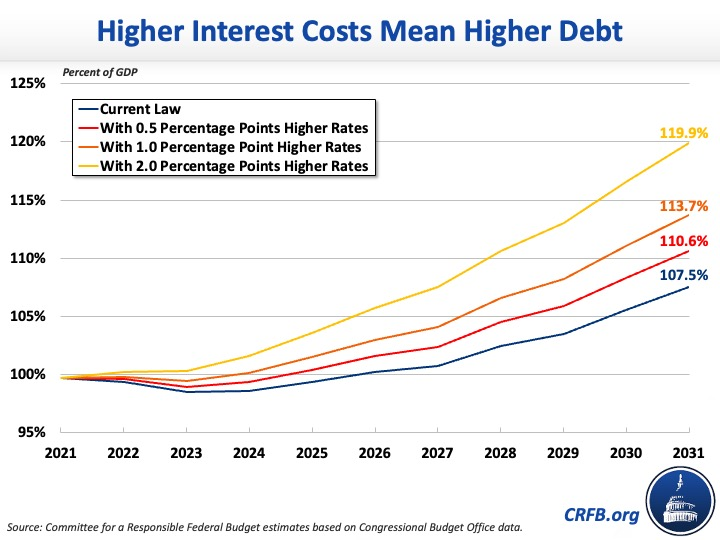# How Would Higher Interest Rates Affect Interest Payments?

Interest on the national debt is projected to be the fastest growing part of the federal budget. We estimate, based on the Congressional Budget Office's (CBO) July 2021 economic forecast, the federal government will spend an average of \$545 billion per year on interest payments and interest costs will total \$5.5 trillion between Fiscal Years (FY) 2022 and 2031 under current law. If interest rates rise faster than projected in CBO's forecast, interest costs would be even higher. In this analysis we show that:

• If interest rates are 50 basis points (0.5 percentage points) higher than projected, average annual interest costs would increase by \$94 billion per year. Interest payments would total \$6.4 trillion over the FY 2022 to 2031 period, and federal deficits would increase (with revenue effects) by \$105 billion per year.
• If interest rates are 100 basis points (1.0 percentage point) higher, average annual interest costs would increase by \$187 billion. Interest payments would total \$7.3 trillion over the FY 2022 to 2031 period, and federal deficits would increase (with revenue effects) by \$209 billion per year.
• If interest rates are 200 basis points (2.0 percentage points) higher, average annual interest costs would increase by \$375 billion. Interest payments would total \$9.2 trillion over the FY 2022 to 2031 period, and federal deficits would increase (with revenue effects) by \$418 billion per year
• Interest rates that are two percentage points above CBO's forecast could push debt as a share of Gross Domestic Product (GDP) up to a record 120 percent by FY 2031 and increase ten-year budget deficits by up to \$4.2 trillion.

### Higher Interest Rates Would Further Increase Interest Costs

At 1.9 percent, the average interest rate on ten-year Treasury notes is slightly above the 1.8 percent CBO projected it would be this quarter. While interest rate projections are highly uncertain, should they continue to outperform CBO’s forecast, the result will be more debt and more spending needed to service that debt.Under current law, we project interest spending will total \$5.5 trillion between FY 2022 and 2031 – an average of \$545 billion per year. If interest rates on the projected annual debt stock are 50 basis points (0.5 percentage points) higher than what CBO currently projects – reflecting a continuation of the current disparity between forecasts and reality – interest costs would increase by an average of \$94 billion per year and interest costs would total \$6.4 trillion through FY 2031. If interest rates are 100 basis points (one full percentage point) above CBO’s forecast, interest costs would be an average of \$187 billion per year higher and total \$7.3 trillion over the FY 2022 to 2031 period.

If rates increase further and are 200 basis points (two percentage points) above CBO’s forecast, interest costs would increase by an average of \$375 billion per year and interest costs would total \$9.2 trillion through FY 2031.

### Higher Interest Rates Would Drive Up Deficits and Debt

Higher interest costs would increase annual budget deficits and the national debt. Under current law, we estimate budget deficits will total \$12.7 trillion over the FY 2022 to 2031 period. Including the revenue effects and higher interests costs that would accompany higher rates, budget deficits would be \$1.0 trillion larger if interest rates are 0.5 percentage points higher. With rates that are one or two percentage points higher, deficits would be \$2.1 trillion or \$4.2 trillion larger, respectively, through FY 2031.

Under current law, debt is projected to reach a record 107.5 percent of GDP by FY 2031. If interest rates are 50 basis points higher, debt would reach 110.6 percent of GDP by 2031. Should rates increase by 100 or 200 basis points, debt would total 113.7 percent of GDP or 119.9 percent of GDP, respectively, by FY 2031.As the national debt continues to grow and interest rates on Treasury bills rise from historically low levels, the federal government's interest payments on its debt are projected to grow rapidly through FY 2031. In turn, interest will comprise a larger and larger share of federal spending, increasing deficits and crowding out other important priorities in the federal budget. Policymakers should work to put debt on a sustainable path through a combination of entitlement reforms, wasteful spending reductions, and tax revenue increases to put debt and deficits on a more sustainable path.